This article was published as a part of the Data Science Blogathon

## Introduction

In my previous article, I talk about the theoretical concepts about outliers and trying to find the answer to the question: “When we have to drop outliers and when to keep outliers?”.

To gain a better understanding of this article, firstly you have to read that and then proceed with this so that you have a clear idea about the outlier analysis in Data Science Projects.

In this article, we will try to give the answer to the following questions along with the Python implementation,

👉 How to treat outliers?

👉 How to detect outliers?

👉 What are the techniques for outlier detection and removal?

## Let’s get started

### How to treat outliers?

👉 Trimming: It excludes the outlier values from our analysis. By applying this technique our data becomes thin when there are more outliers present in the dataset. Its main advantage is its fastest nature.

👉Capping: In this technique, we cap our outliers data and make the limit i.e, above a particular value or less than that value, all the values will be considered as outliers, and the number of outliers in the dataset gives that capping number.

For Example, if you’re working on the income feature, you might find that people above a certain income level behave in the same way as those with a lower income. In this case, you can cap the income value at a level that keeps that intact and accordingly treat the outliers.

👉Treat outliers as a missing value: By assuming outliers as the missing observations, treat them accordingly i.e, same as those of missing values.

You can refer to the missing value article

👉 Discretization: In this technique, by making the groups we include the outliers in a particular group and force them to behave in the same manner as those of other points in that group. This technique is also known as Binning.

### How to detect outliers?

👉 For Normal distributions: Use empirical relations of Normal distribution.

– The data points which fall below mean-3*(sigma) or above mean+3*(sigma) are outliers.

where mean and sigma are the average value and standard deviation of a particular column.Fig. Characteristics of a Normal Distribution

Image Source:

👉 For Skewed distributions: Use Inter-Quartile Range (IQR) proximity rule.

– The data points which fall below Q1 – 1.5 IQR or above Q3 + 1.5 IQR are outliers.

where Q1 and Q3 are the 25th and 75th percentile of the dataset respectively, and IQR represents the inter-quartile range and given by Q3 – Q1.Fig. IQR to detect outliers

Image Source:

👉 For Other distributions: Use percentile-based approach.

For Example, Data points that are far from 99% percentile and less than 1 percentile are considered an outlier.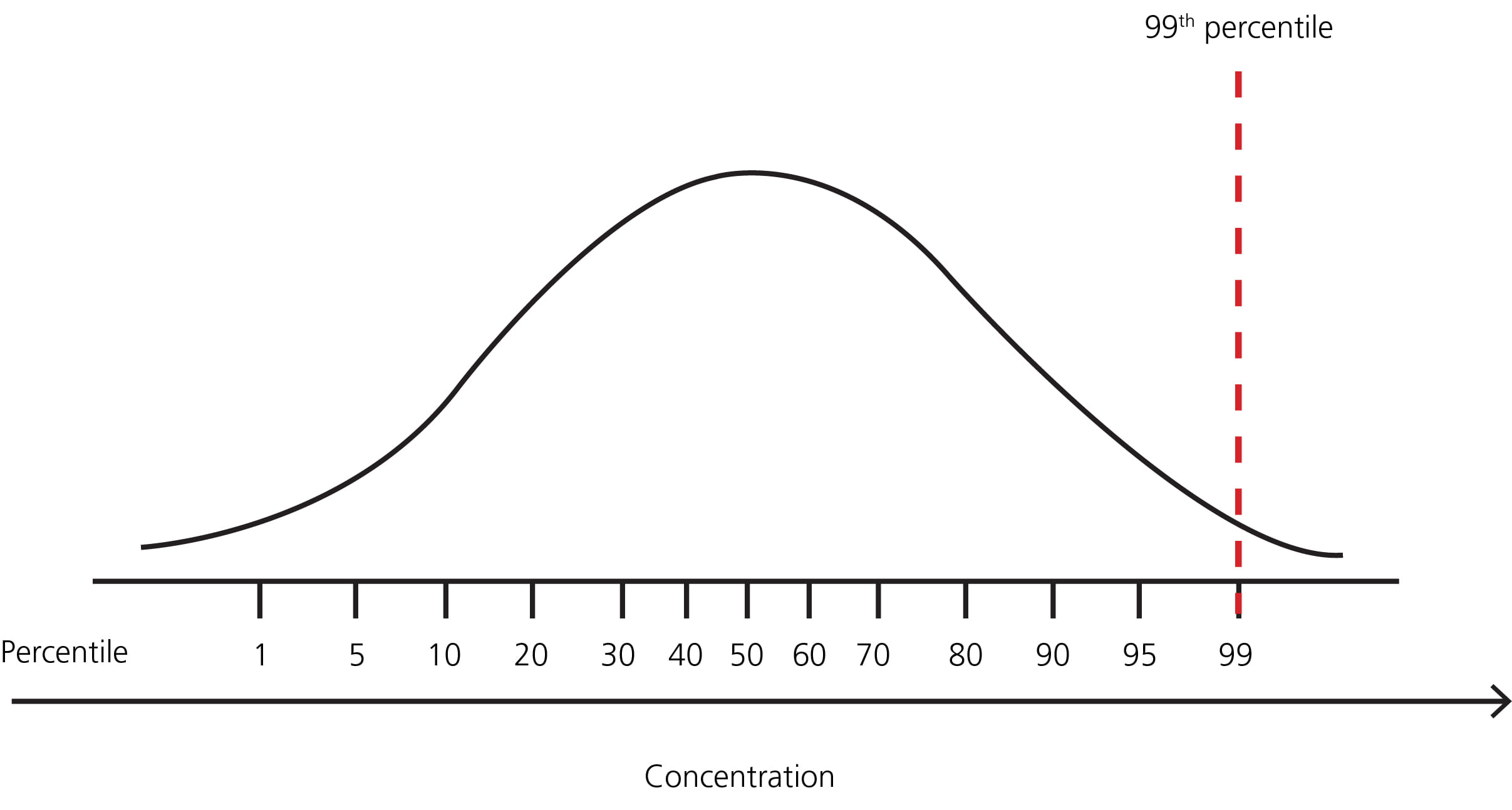Fig. Percentile representation

Image Source:

### Techniques for outlier detection and removal:

👉 Z-score treatment :

Assumption– The features are normally or approximately normally distributed.

Step-1: Importing Necessary Dependencies

```import numpy as np
import pandas as pd
import matplotlib.pyplot as plt
import seaborn as sns```

```df = pd.read_csv('placement.csv')
df.sample(5)```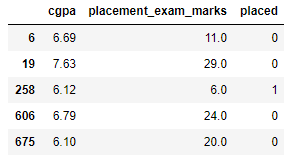Step-3: Plot the Distribution plots for the features

```import warnings
warnings.filterwarnings('ignore')
plt.figure(figsize=(16,5))
plt.subplot(1,2,1)
sns.distplot(df['cgpa'])
plt.subplot(1,2,2)
sns.distplot(df['placement_exam_marks'])
plt.show()```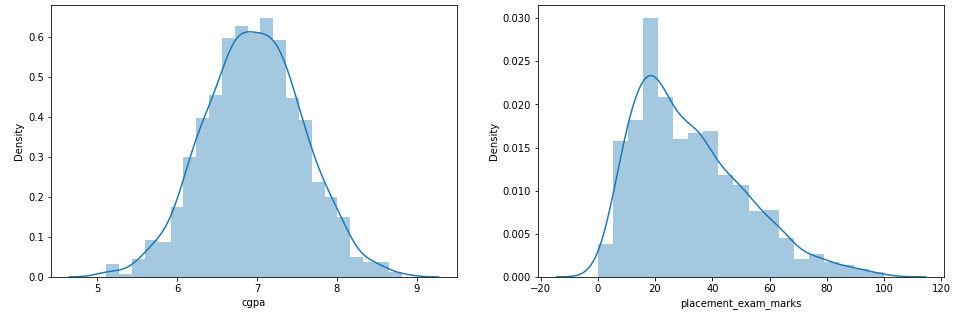Step-4: Finding the Boundary Values

```print("Highest allowed",df['cgpa'].mean() + 3*df['cgpa'].std())
print("Lowest allowed",df['cgpa'].mean() - 3*df['cgpa'].std())```

Output:

```Highest allowed 8.808933625397177
Lowest allowed 5.113546374602842```

Step-5: Finding the Outliers

`df[(df['cgpa'] > 8.80) | (df['cgpa'] < 5.11)]`

Step-6: Trimming of Outliers

```new_df = df[(df['cgpa'] < 8.80) & (df['cgpa'] > 5.11)]
new_df```

Step-7: Capping on Outliers

```upper_limit = df['cgpa'].mean() + 3*df['cgpa'].std()
lower_limit = df['cgpa'].mean() - 3*df['cgpa'].std()```

Step-8: Now, apply the Capping

```df['cgpa'] = np.where(
df['cgpa']>upper_limit,
upper_limit,
np.where(
df['cgpa']<lower_limit,
lower_limit,
df['cgpa']
)
)```

Step-9: Now see the statistics using “Describe” Function

`df['cgpa'].describe()`

Output:

```count    1000.000000
mean        6.961499
std         0.612688
min         5.113546
25%         6.550000
50%         6.960000
75%         7.370000
max         8.808934
Name: cgpa, dtype: float64```

This completes our Z-score based technique!

👉 IQR based filtering :

Used when our data distribution is skewed.

Step-1: Import necessary dependencies

```import numpy as np
import pandas as pd
import matplotlib.pyplot as plt
import seaborn as sns```

```df = pd.read_csv('placement.csv')

Step-3: Plot the distribution plot for the features

```plt.figure(figsize=(16,5))
plt.subplot(1,2,1)
sns.distplot(df['cgpa'])
plt.subplot(1,2,2)
sns.distplot(df['placement_exam_marks'])
plt.show()```

Step-4: Form a Box-plot for the skewed feature

`sns.boxplot(df['placement_exam_marks'])`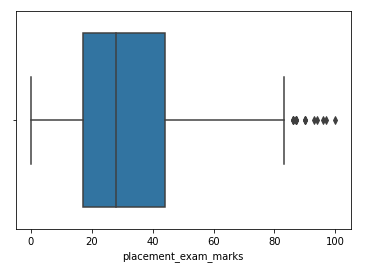Step-5: Finding the IQR

```percentile25 = df['placement_exam_marks'].quantile(0.25)
percentile75 = df['placement_exam_marks'].quantile(0.75)```

Step-6: Finding upper and lower limit

```upper_limit = percentile75 + 1.5 * iqr
lower_limit = percentile25 - 1.5 * iqr```

Step-7: Finding Outliers

```df[df['placement_exam_marks'] > upper_limit]
df[df['placement_exam_marks'] < lower_limit]```

Step-8: Trimming

```new_df = df[df['placement_exam_marks'] < upper_limit]
new_df.shape```

Step-9: Compare the plots after trimming

```plt.figure(figsize=(16,8))
plt.subplot(2,2,1)
sns.distplot(df['placement_exam_marks'])
plt.subplot(2,2,2)
sns.boxplot(df['placement_exam_marks'])
plt.subplot(2,2,3)
sns.distplot(new_df['placement_exam_marks'])
plt.subplot(2,2,4)
sns.boxplot(new_df['placement_exam_marks'])
plt.show()```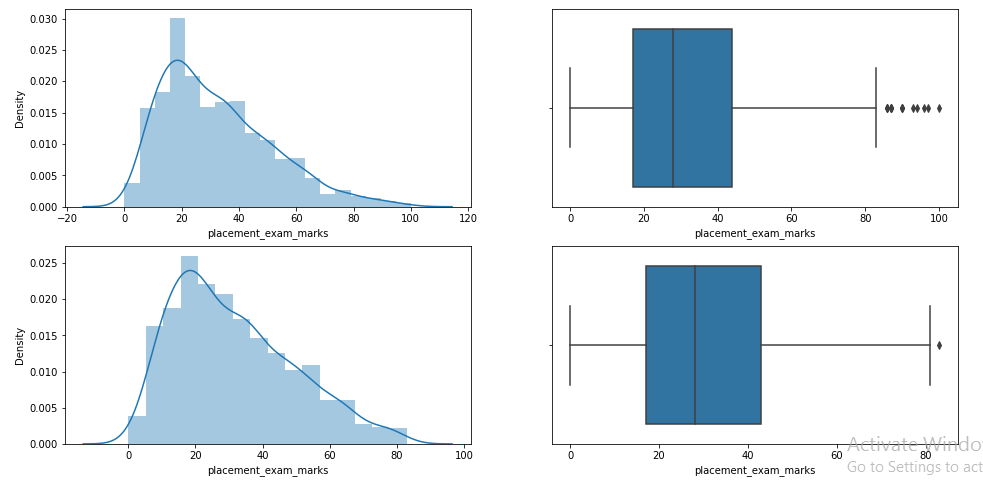Step-10: Capping

```new_df_cap = df.copy()
new_df_cap['placement_exam_marks'] = np.where(
new_df_cap['placement_exam_marks'] > upper_limit,
upper_limit,
np.where(
new_df_cap['placement_exam_marks'] < lower_limit,
lower_limit,
new_df_cap['placement_exam_marks']
)
)```

Step-11: Compare the plots after capping

```plt.figure(figsize=(16,8))
plt.subplot(2,2,1)
sns.distplot(df['placement_exam_marks'])
plt.subplot(2,2,2)
sns.boxplot(df['placement_exam_marks'])
plt.subplot(2,2,3)
sns.distplot(new_df_cap['placement_exam_marks'])
plt.subplot(2,2,4)
sns.boxplot(new_df_cap['placement_exam_marks'])
plt.show()```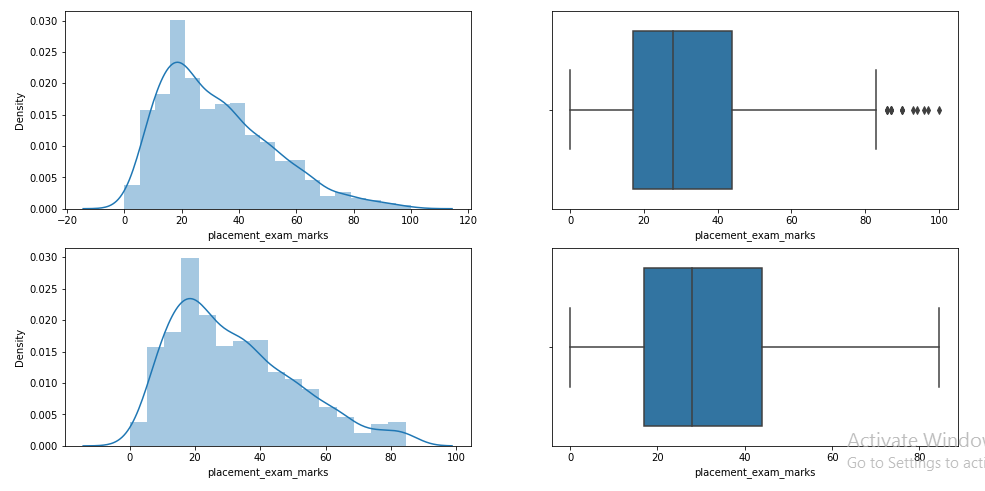This completes our IQR based technique!

👉 Percentile :

– This technique works by setting a particular threshold value, which decides based on our problem statement.

– While we remove the outliers using capping, then that particular method is known as Winsorization.

– Here we always maintain symmetry on both sides means if remove 1% from the right then in the left we also drop by 1%.

Step-1: Import necessary dependencies

```import numpy as np
import pandas as pd```

```df = pd.read_csv('weight-height.csv')
df.sample(5)```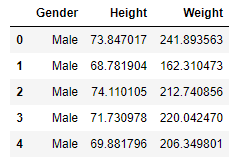Step-3: Plot the distribution plot of “height” feature

`sns.distplot(df['Height'])`

Step-4: Plot the box-plot of “height” feature

`sns.boxplot(df['Height'])`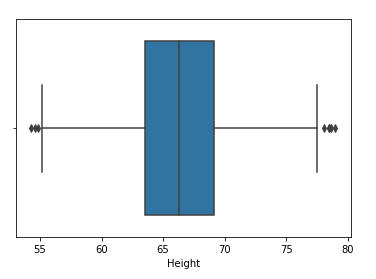Step-5: Finding upper and lower limit

```upper_limit = df['Height'].quantile(0.99)
lower_limit = df['Height'].quantile(0.01)```

Step-7: Apply trimming

`new_df = df[(df['Height'] <= 74.78) & (df['Height'] >= 58.13)]`

Step-8: Compare the distribution and box-plot after trimming

```sns.distplot(new_df['Height'])
sns.boxplot(new_df['Height'])```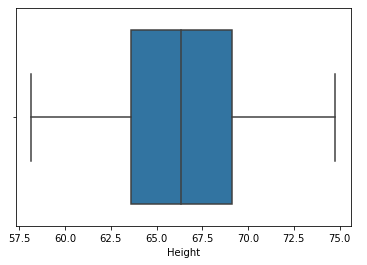👉 Winsorization :

Step-9: Apply Capping(Winsorization)

```df['Height'] = np.where(df['Height'] >= upper_limit,
upper_limit,
np.where(df['Height'] <= lower_limit,
lower_limit,
df['Height']))```

Step-10: Compare the distribution and box-plot after capping

```sns.distplot(df['Height'])
sns.boxplot(df['Height'])```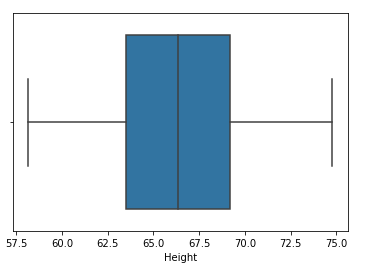This completes our percentile-based technique!

## End Notes

If you liked this and want to know more, go visit my other articles on Data Science and Machine Learning by clicking on the Link

Something not mentioned or want to share your thoughts? Feel free to comment below And I’ll get back to you.

## Chirag Goyal

Currently, I am pursuing my Bachelor of Technology (B.Tech) in Computer Science and Engineering from the Indian Institute of Technology Jodhpur(IITJ). I am very enthusiastic about Machine learning, Deep Learning, and Artificial Intelligence.

The media shown in this article are not owned by Analytics Vidhya and is used at the Author’s discretion.